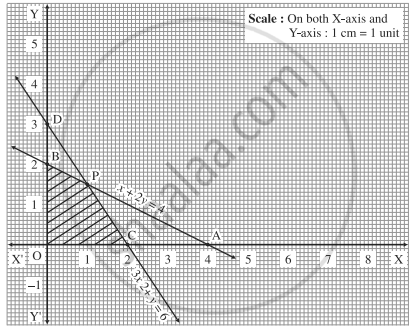# A company produces two types of articles A and B which require silver and gold. Each unit of A requires 3 gm of silver and 1 gm of gold, while each unit of B requires 2 gm of silver and 2 gm of gold. - Mathematics and Statistics

Sum

A company produces two types of articles A and B which require silver and gold. Each unit of A requires 3 gm of silver and 1 gm of gold, while each unit of B requires 2 gm of silver and 2 gm of gold. The company has 6 gm of silver and 4 gm of gold. Construct the inequations and find feasible solution graphically

#### Solution

Let the company produces x units of article A and y units of article B.
The given data can be tabulated as:

 Article A(x) Article B(y) Availability Gold 1 2 4 Silver 3 2 6

Inequations are:

x + 2y ≤ 4 and 3x + 2y ≤ 6

x and y are number of items, x ≥ 0, y ≥ 0

First we draw the lines AB and CD whose equations are x + 2y = 4 and 3x + 2y = 6 respectively.

 Line Equation Points on the X-axis Points on the Y-axis Sign Region AB x +2y = 4 A(4, 0) B(0, 2) ≤ origin side of line AB CD 3x + 2y = 6 C(2, 0) D(0, 3) ≤ origin side of line CDThe feasible solution is OCPBO which is shaded in the graph.

Concept: Linear Programming Problem (L.P.P.)
Is there an error in this question or solution?
Chapter 7: Linear Programming - Exercise 7.2 [Page 234]

Share